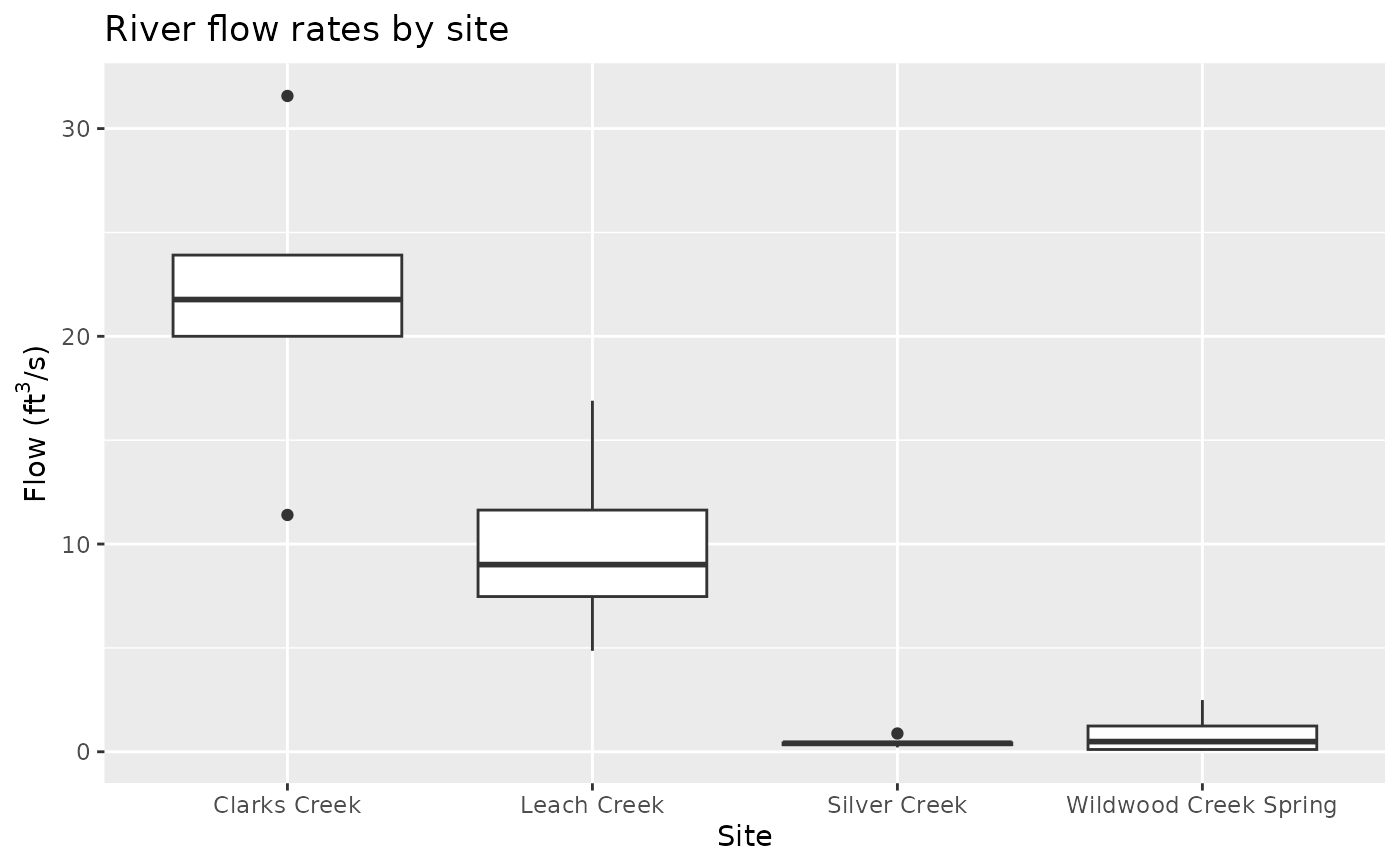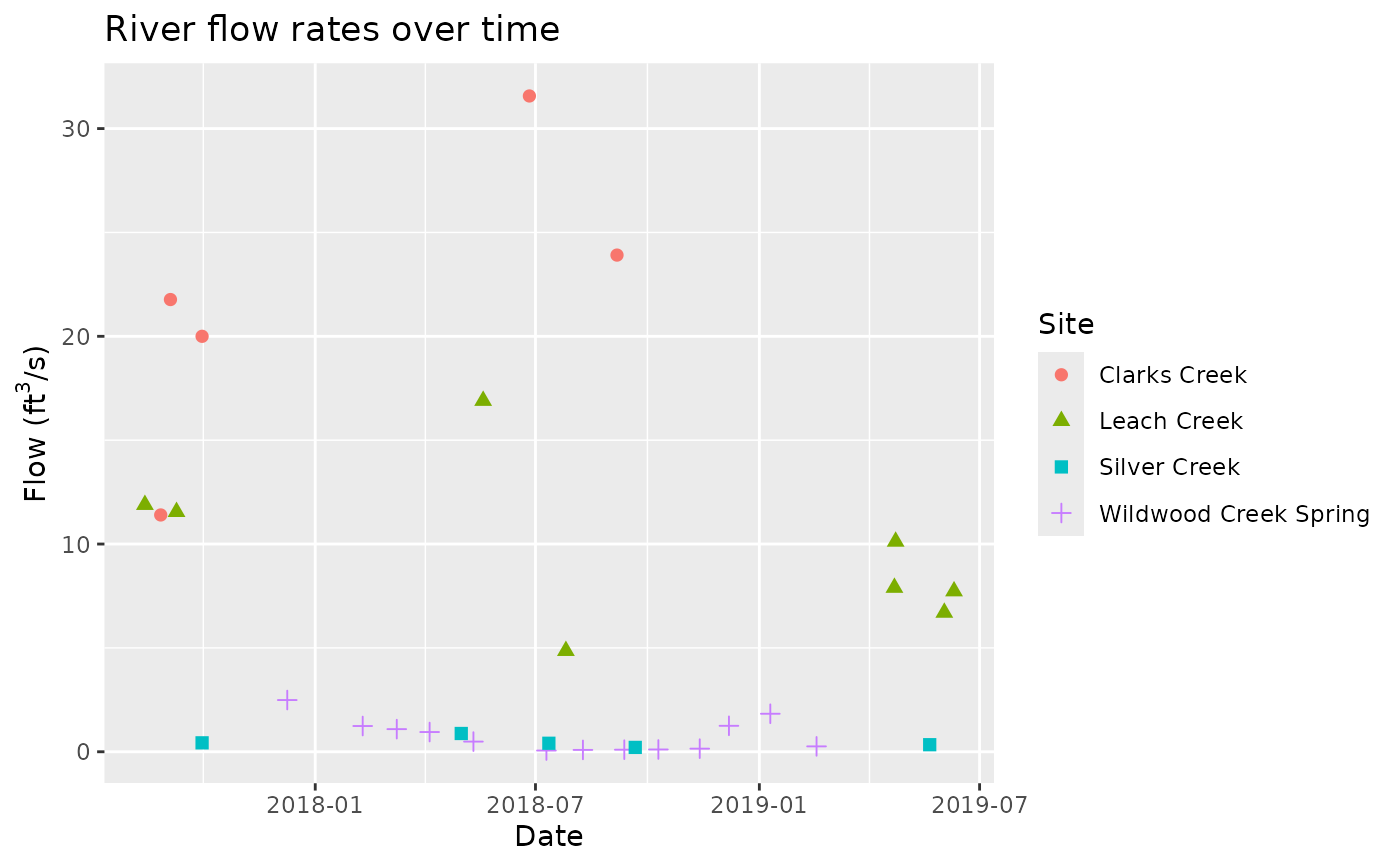Flow rates (mesured in cubic feet per second) of Clarks Creek, Leach Creek, Silver Creek, and Wildwood Creek Spring collected by volunteers of the Pierce Conservation District in the State of Washington in the US.

## Usage

flow_rates

## Format

A data frame with 31 rows and 3 variables.

site

Location where measurements were taken.

date

Date measurements were taken.

flow

Flow rate of the river in cubic feet per second.

## Examples


library(ggplot2)

# River flow rates by site
ggplot(flow_rates, aes(x = site, y = flow)) +
geom_boxplot() +
labs(
title = "River flow rates by site",
x = "Site",
y = expression(paste("Flow (ft"^3 * "/s)"))
)# River flow rates over time
ggplot(flow_rates, aes(x = date, y = flow, color = site, shape = site)) +
geom_point(size = 2) +
labs(
title = "River flow rates over time",
x = "Date",
y = expression(paste("Flow (ft"^3 * "/s)")),
color = "Site", shape = "Site"
)